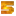Endurance is a skill in Modern Combat 5: Blackout

## Modern Combat 5: Blackout

Endurance is the sixth skill in the Recon soldier class.

{{#infoboxbuilder: | 0:Theme = default | 0:CustomModule = | 0:MainImageCaption = | 0:ToggleContentLongerThan = 1000

| 1:Type = Title

```| 1:Value = Endurance
```

| 2:Type = MainImage

| 2:Value =

| 3:Type = Line

```| 3:Label = Effect
| 3:Value = Movement speed while carrying primary weapon is increased
```

| 4:Type = Line

```| 4:Label = Duration
| 4:Value =
```

| 5:Type = Line

```| 5:Label = Specialization
| 5:Value =
```

| 6:Type = Line

```| 6:Label = Class
| 6:Value = Support
```

| 7:Type = Line

```| 7:Label = Tier
| 7:Value =
```

| 8:Type = Line

```| 8:Label = Unlocked
| 8:Value =
```

| 9:Type = Line

```| 9:Label = Cost
| 9:Value = 6 SP (Rank 1); 5 SP (Rank2)
```

| 10:Type = Title

```| 10:Value =
```

| 11:Type = Image

```| 11:Value =
```

| 12:Type = Line

```| 12:Label = Effect
| 12:Value =
```

| 13:Type = Line

```| 13:Label = Unlocked
| 13:Value =
```

| 14:Type = Line

```| 14:Label = Cost
| 14:Value =
```

| 15:Type = Title

```| 15:Value =
```

| 16:Type = Image

```| 16:Value =
```

| 17:Type = Line

```| 17:Label = Effect
| 17:Value =
```

| 18:Type = Line

```| 18:Label = Unlocked
| 18:Value =
```

| 19:Type = Line

```| 19:Label = Cost
| 19:Value =

```

| 20:Type = Title

```| 20:Value =
```

| 21:Type = Image

```| 21:Value =
```

| 22:Type = Line

```| 22:Label = Effect
| 22:Value =
```

| 23:Type = Line

```| 23:Label = Unlocked
| 23:Value =
```

| 24:Type = Line

```| 24:Label = Cost
| 24:Value =
```

}}

Rank 1
Movement speed is increased slightly while carrying LMGs
Rank 2
Movement speed is increased moderately while carrying LMGs